Courses

# Test Level 1: Equilibrium

## 25 Questions MCQ Test Chemistry for JEE | Test Level 1: Equilibrium

Description
This mock test of Test Level 1: Equilibrium for JEE helps you for every JEE entrance exam. This contains 25 Multiple Choice Questions for JEE Test Level 1: Equilibrium (mcq) to study with solutions a complete question bank. The solved questions answers in this Test Level 1: Equilibrium quiz give you a good mix of easy questions and tough questions. JEE students definitely take this Test Level 1: Equilibrium exercise for a better result in the exam. You can find other Test Level 1: Equilibrium extra questions, long questions & short questions for JEE on EduRev as well by searching above.
QUESTION: 1

### If we place solid iodine in a closed vessel, after sometime the vessel gets filled up with violet vapour. When equilibrium is attained, the intensity of colour will be

Solution:

since at eqm conc of reactant and product doesnot change therefore intensity of color did not change.​

QUESTION: 2

### A particular ratio of product to reactant helps in predicting the direction in which a given reaction will proceed at any stage. is called

Solution:

The particular ratio is called as reaction quotient which is compared to a given k value and then the direction of equilibrium iis decided.

QUESTION: 3

### 0.023 g of sodium metal is reacted with 100 cm3 of water. The pH of the resulting solution is ______.

Solution: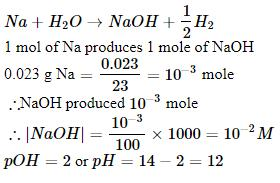QUESTION: 4

BF3does not have proton but still acts as an acid and reacts with NH3. Why is it so? What type of bond is formed between the two?

Solution:

BF3 is an electron deficient compound. Hence, it acts as Lewis acid. NH3 has a lone pair of electrons. Hence, acts as Lewis base. A coordinate bond is formed between the two.
H3N: →BF

QUESTION: 5

pH of a solution of a strong acid is 5.0. What will be the pH of the solution obtained after diluting the given solution a 100 times?

Solution:

Conc. of a strong Acid with pH=5 is 10-5 M. On diluting 100- times the concentration decreases to 10-7.

But the water at 25 degree C will be having 10–7 M of H3O+ ions in solution. So the H3O+ ion concentration in 10-7 M HCl is (10-7+10-7) M

= 2 x 10^-7.

So it pH= - log (2 x 10-7)

= 6.6987

QUESTION: 6

The boiling point of water at atmospheric pressure

Solution:

Boiling Point of water is 100°C

QUESTION: 7

The aqueous solution of sugar does not conduct electricity. However, when sodium chloride is added to water, it conducts electricity. How will you explain this statement on the basis of ionisation and how is it affected by concentration of sodium chloride?

Solution:

• Sugar does not ionise in water but NaCl ionises completely in water and produces Na+ and Cl ions.

• Conductance increases with increase in concentration of salt due to release of more ions.

QUESTION: 8

pH of a saturated solution of Ba(OH)2 is 12. The value of solubility product (Ksp) of Ba(OH)2 is

Solution:

Given, pH of Ba(OH)2 = 12
pOH = 14-pH
= 14-12 = 2
We know that,
pOH = -log [OH-]
2 =-log [OH-]
[OH-] = antilog (-2)
[OH-] = 1 x 10-2

Ba(OH)2dissolves in water as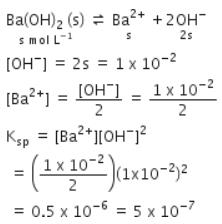QUESTION: 9

0.3 g of Ca(OH)2 is dissolved in water to give 500 mL of solution. The pH of the solution is

Solution:

For 0.3 g of Ca(OH)2 dissolved in water to give 500 mL of solution: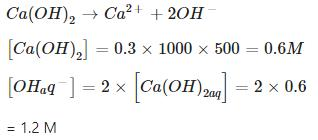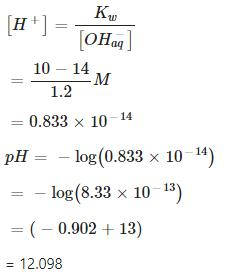QUESTION: 10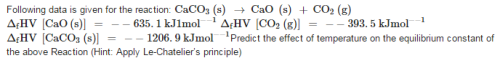Solution:

∆Hreaction = (∆HProduct - ∆Hreactant)
= (-635.1 - 393.5)-(-1206.9) = 178.3 kJ mol-1
So, we can say that the reaction is endothermic in nature.
So, an increase in temperature will drive the reaction in a forward direction. (According to Le Chetelier principle-a principle stating that if a constraint (such as a change in pressure, temperature, or concentration of a reactant) is applied to a system in equilibrium, the equilibrium will shift so as to tend to counteract the effect of the constraint.)

QUESTION: 11

The mass of a gas dissolved in a given mass of a solvent at any temperature is proportional to the pressure of the gas above the solvent.

Solution:

Xsolute (Henrys Law)

QUESTION: 12

In order to decide what course the reaction adopts and make a qualitative prediction about the effect of a change in conditions on equilibrium we use

Solution:

Le Chaterlier principle is used.

QUESTION: 13

Acidity of BF3can be explained on the basis of which of the following concepts?

Solution:

According to Lewis concept, a positively charged or an electron deficient species acts as Lewis acid. BFis an electron deficient compound with B having 6 electrons only.

QUESTION: 14

Calculate the hydrogen ion concentration in the human blood whose pH is 7.38.

Solution:

Human blood, 7.38: pH = 7.38 = – log [H+]
∴ [H+] = 107.38

4.17 × 10–8 M

QUESTION: 15

Does the number of moles of reaction products increase, decrease or remain same when each of the following equilibria is subjected to a decrease in pressure by increasing the volume?

PCl5(g)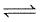PCl3 (g) + Cl2 (g)

Solution:

When pressure is decreased , volume of reaction system will increase. So reaction will move in a direaction where there will be more number of moles. So reaction will move in forward direaction and moles of product will increase.

QUESTION: 16

When the soda bottle is opened, some of the dissolved carbon dioxide gas escapes because of

Solution:

As P decreases solubility of gas also decreases (Henrys Law)​

QUESTION: 17

A catalyst increases the rate of the chemical reaction for the conversion of reactants to products by

Solution:

Catalyst changes the course of reaction by decreasing low Ea energy pathway.

QUESTION: 18

The dynamic nature of chemical equilibrium can be demonstrated in the synthesis of ammonia by Haber.s process. Choose the appropriate option given below

Solution:

Dynamic nature can be studied by deuterium isotope.

QUESTION: 19

The ionization constant of acetic acid is 1.74 x 10−5. Calculate the degree of dissociation of acetic acid in its 0.05 M solution. Calculate the concentration of acetate ion in the solution and its pH.

Solution: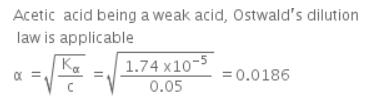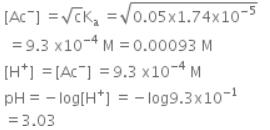QUESTION: 20

Does the number of moles of reaction products increase, decrease or remain same when each of the following equilibria is subjected to a decrease in pressure by increasing the volume?

PCl5(g)PCl3 (g) + Cl2 (g)

Solution:

The number of moles of reaction products will increase. According to Le Chatelier’s principle, if pressure is decreased, then the equilibrium shifts in the direction in which the number of moles of gases is more. In the given reaction, the number of moles of gaseous products is more than that of gaseous reactants. Thus, the reaction will proceed in the forward direction. As a result, the number of moles of reaction products will increase.

QUESTION: 21

For liquid ⇌vapour equilibrium, at a given temperature, the constant is

Solution:

vapour pressure at the given temperature is constant.​

QUESTION: 22

A bare proton, H+ is very reactive and cannot exist freely in aqueous solutions. Thus, it bonds to the oxygen atom of a solvent water molecule to give

Solution:

H+ attacks on one lone pair of H2O to form H3O+ which is more stable than H+.

QUESTION: 23

If, in the reaction N2O4⇌2NO2, x is that part of N2O4which dissociates, then the number of molecules at equilibrium will be

Solution: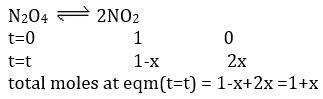QUESTION: 24

The ionization constant of HF is 3.2 x10−4.Calculate the degree of dissociation of HF in its 0.02 M solution. Calculate the concentration of all species present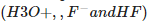in the solution and its H

Solution: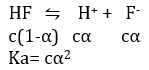QUESTION: 25

What will be the correct order of vapour pressure of water, acetone and ether At 30∘C. Given that among these compounds, water has maximum boiling point and ether has minimum boiling point?

Solution:

Higher the boiling point lower will be the vapour pressure. Thus, the correct order of vapour pressure is Water < Acetone < Ether.